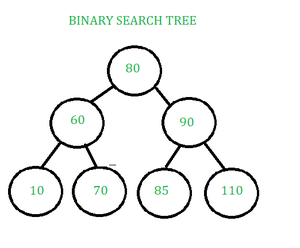GFG App
Open AppBrowser
Continue

Binary Search Tree (BST) is a special binary tree that has the properties:

•  The left subtree contains only the keys which are lesser than the key of the node.
•  The right subtree contains only the keys which are greater than the key of the node.
• The left and right subtree both should be binary search tree.BINARY SEARCH TREE

Operations on Binary Search tree:

The four basic operations of BST:

1. Searching,
2. Insertion, and
3. Deletion
4. Traversals

1. Searching in a BST:

Searching in BST involves the comparison of the key values. If the key value is equal to root key then, search successful, if lesser than root key then search the key in the left subtree and if the key is greater than root key then search the key in the right subtree.

Searching in BST algorithm:-

• Check if tree is NULL, if the tree is not NULL then follow the following steps.
• Compare the key to be searched with the root of the BST.
• If the key is lesser than the root then search in the left subtree.
• If the key is greater than the root then search in the right subtree.
• If the key is equal to root then, return and print search successful.
• Repeat step 3, 4 or 5 for the obtained subtree.

2. Insertion in a BST:

Insertion in BST involves the comparison of the key values. If the key value is lesser than or equal to root key then go to left subtree, find an empty space following to the search algorithm and insert the data and if the key is greater than root key then go to right subtree, find an empty space following to the search algorithm and insert the data.

3. Deletion in a BST:

Deletion in BST involves three cases:-

First, search the key to be deleted using searching algorithm and find the node. Then, find the number of children of the node to be deleted.

• Case 1- If the node to be deleted is leaf node: If the node to be deleted is a leaf node, then delete it.
• Case 2- If the node to be deleted has one child: If the node to be deleted has one child then, delete the node and place the child of the node at the position of the deleted node.
• Case 3- If the node to be deleted has two children: If the node to be deleted has two children then, find the inorder successor or inorder predecessor of the node according to the nearest capable value of the node to be deleted. Delete the inorder successor or predecessor using the above cases. Replace the node with the inorder successor or predecessor.

4. Traversals in a BST:

There are 4 types of traversals of the Binary Search Tree.

Level Order Traversal: Each node of the tree is traversed level by level in order of its appearance.

Pre-order Traversal: The nodes are traversed in the format of root and then left subtree and then right subtree.

Inorder Traversal: The nodes are traversed in the format of left subtree and then root and then right subtree.

Post Traversal: The nodes are traversed in the format of left subtree  and then right subtree and then root

Applications of Binary Search tree:

• BSTs are used for indexing.
• It is also used to implement various searching algorithms.
• IT can be used to implement various data structures.
• BSTs can be used in decision support systems to store and quickly retrieve data.
• BSTs can be used to store and quickly retrieve data in computer simulations.
• BSTs can be used to implement fast autocomplete systems.
• BSTs can be used to implement decision trees, which are used in machine learning and artificial intelligence to model decisions and predict outcomes. Decision trees are used in many applications, including medical diagnosis, financial analysis, and marketing research.
• BSTs can be used in encryption algorithms such as RSA, which is a public-key encryption algorithm used in secure communication protocols. RSA uses a BST to generate public and private keys.
• BSTs can be used to compress data by storing frequently occurring values in a smaller space and less frequently occurring values in a larger space. This technique is used in many applications, including image and audio compression, data transmission, and file compression.

Real-time Application of Binary Search tree:

• BSTs are used for indexing in databases.
• It is used to implement searching algorithms.
• BSTs are used to implement Huffman coding algorithm.
• It is also used to implement dictionaries.
• Used for data caching.
• Used in Priority queues.
• Used in spell checkers.

• BST is fast in insertion and deletion when balanced. It is fast with a time complexity of O(log n).
• BST is also for fast searching, with a time complexity of O(log n) for most operations.
• BST is efficient. It is efficient because they only store the elements and do not require additional memory for pointers or other data structures.
• We can also do range queries – find keys between N and M (N <= M).
• BST code is simple as compared to other data structures.
• BST can automatically sort elements as they are inserted, so the elements are always stored in a sorted order.
• BST can be easily modified to store additional data or to support other operations. This makes it flexible.